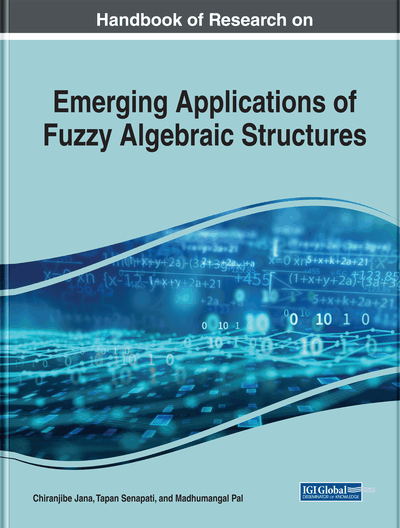Some Types of Ideals in QI-Algebras

Ravikumar Bandaru (GITAM University (Deemed), Hyderabad, India)
DOI: 10.4018/978-1-7998-0190-0.ch016
Available
\$37.50
No Current Special Offers

Abstract

In this chapter, ideals of QI-algebra are considered. Given a subset of a right distributive QI-algebra, the smallest ideal containing it is constructed. Also, the notions of implicative ideal, fantastic ideal, and normal ideal in a right distributive QI-algebra are introduced, and the authors proved that these notions are equivalent.
Chapter Preview
Top

2. Preliminaries

First, we recall certain definitions and properties from Saeid et al.(2017), Abbott (1967) & Chen et.al. (1995) that are required in the paper.

Definition 2.1 (Iseki, 1980) A BCI-algebra is an algebra (X,*,0) of type (2,0) satisfying the following conditions:

• (1)

(x*y)*(x*z)≤(z*y)

• (2)

x*(x*y)≤y

• (3)

xx

• (4)

xy and yy imply x=y

• (5)

x≤0 implies x=0

where xy is defined by x*y=0.

If (5) is replaced by (6) 0≤x, then the algebra is called a BCK-algebra. It is known that every BCK-algebra is a BCI-algebra but not conversely. A BCK-algebra satisfying the property x*(y*x)= x for all x,yX is called an implicative BCK-algebra.

Several generalizations of a BCK-algebra, in the form of definitions, one can see in the paper (Saeid et al., 2017).

Definition 2.2 (Abbott, 1967) A groupoid (X,*) is called an implication algebra if it satisfies the following identities:

• (a)

(x*y)*x=x

• (b)

(x*y)*y=(y*x)*x

• (c)

X*(y*z)=y*(x*z)

for all x,y,zX.

Definition 2.3 (Abbott, 1967) Let (X,*) be an implication algebra and binary operation “” on X be defined byThenis said to be a dual implication algebra. In fact, the axioms of that are as follows:

for all x,y,zX.

Chen & Oliveira (1995) proved that in any implication algebra (X,*) the identity x*y=y*y holds for all x,yX. We denote the identity x*x=y*y by the constant 0. The notion of BI-algebras comes from the (dual) implication algebra.

Complete Chapter List

Search this Book:
Reset